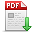# 李娜娜,黄琨强,张秋闻,等.基于自适应量化器选择的编码率失真优化判决算法[J].郑州大学学报(工学版),2019,40(03):4. 　Rate Distortion Optimization Decision Algorithm of Coding Based on Adaptive Quantizer Selection[J].Journal of Zhengzhou University (Engineering Science),2019,40(03):4. 点击复制 基于自适应量化器选择的编码率失真优化判决算法() 分享到： var jiathis_config = { data_track_clickback: true };

40

2019年03期

4

2019-04-30

## 文章信息/Info

Title:
Rate Distortion Optimization Decision Algorithm of Coding Based on Adaptive Quantizer Selection

A

Abstract:
In order to solve the problem of high complexity of HEVC coding, an efficient adaptive quantizer selection and rate distortion optimized quantization decision algorithm is proposed in this paper. The quantizer determines whether the quantization level of the transform coefficient is equal or zero and whether the sum of quantization levels is less than the set threshold.If the quantization level is equal or zero, the traditional RDOQ is used directly.Otherwise, a threshold decision is made,and finally determine the optimal quantizer.The proposed decision algorithm can select two quantization methods: Uniform Scalar Quantizer with small computational complexity and RDOQ with low bit rate self-adaptively, and the computational complexity of the encoder is reduced.The experimental results shows that our proposed algorithm can save about 10.52% of coding time.